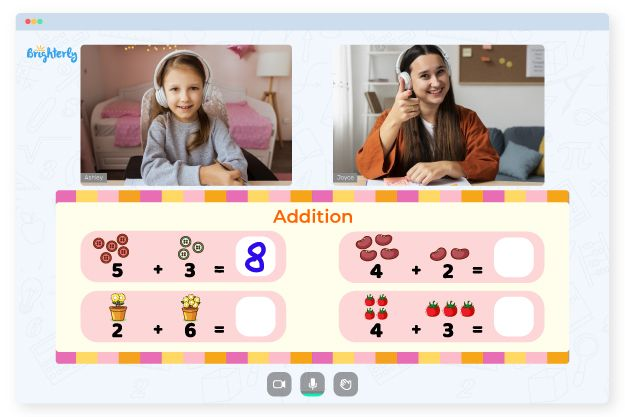Grade school students use different approaches to learning addition. The choice of a method depends on how many digits there are in numbers they are learning to add. For mastering one-digit addition, tutors teach to use hands, but when there are three digits, more complex rules are followed.

Kids can use a 3 digit addition worksheet as a learning resource. Worksheets contain addition tasks, word questions, and other related exercises. This article will give more details about these worksheets so that you can decide if you will introduce them to your students.

## How Do Three Digits Addition Worksheets Work?

A 3-digit addition worksheet offers to add three-digit numbers by either grouping or ungrouping them using some basic addition rules. To be able to add three digits, you should insert the numbers in the column based on the current value of each digit, such as 1, 10, 100, and 1000. The numbers added are called multipliers, while the answer obtained by adding the numbers is called the total.

Math for Kids

Is Your Child Struggling With Math?
1:1 Online Math Tutoring## Benefits of a 3 Digit Addition Worksheet

Kids can perform better in arithmetic operations and speed by learning how to add 3-digit numbers using worksheets. The most effective method to learn is to answer several questions on the same topic. The three-digit addition worksheets present the kids with varying challenges to achieve the goal.### 3 Digit Addition Worksheets PDF### 3 Digit Addition Worksheets PDF### 3 Digit Addition Worksheets PDF### 3 Digit Addition Worksheets PDF

3 Digit Addition With Regrouping Free Worksheets

The 3 digit addition with regrouping free worksheets are well-designed so that the kids will get more clarity as they move through the levels. Students can work independently, without pressure, and at their own pace.• Try learning with an online tutor.

Does your child need assistance with addition? An online tutor could be of help.